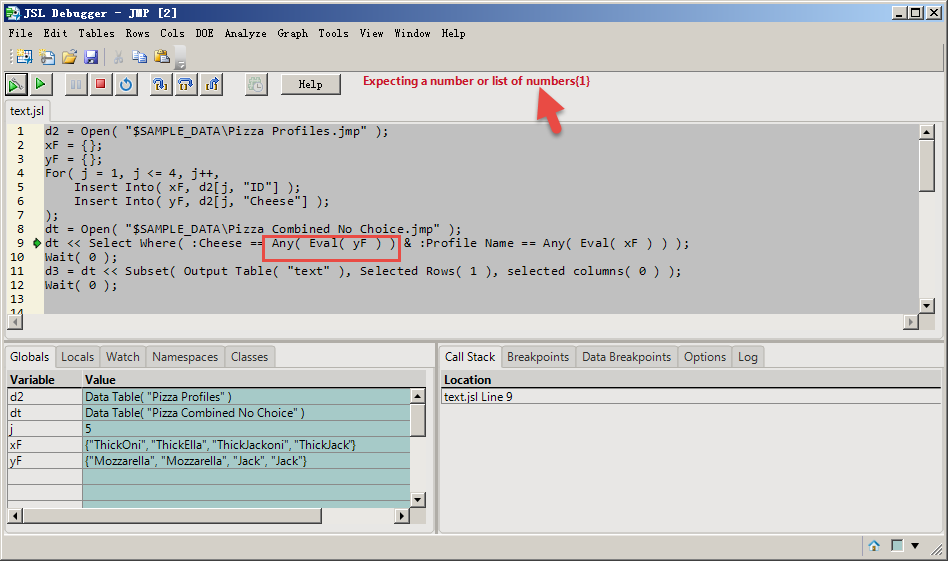Choose Language Hide Translation Bar
Highlighted

## Are there efficient functions or JSL for this choice?

Hello, everyone!

For example, select operations like these two files:
This is true if each of the two columns contains a keyword.
Can a function like "ANY()" match the text?

Thanks!``````d2 = Open( "\$SAMPLE_DATA\Pizza Profiles.jmp" );
xF = {};
yF = {};
For( j = 1, j <= 4, j++,
Insert Into( xF, d2[j, "ID"] );
Insert Into( yF, d2[j, "Cheese"] );
);
dt = Open( "\$SAMPLE_DATA\Pizza Combined No Choice.jmp" );
dt << Select Where( :Cheese ==  Any( Eval( yF ) ) & :Profile Name ==  Any( Eval( xF ) ) );
Wait( 0 );
d3 = dt << Subset( Output Table( "text" ), Selected Rows( 1 ), selected columns( 0 ) );
Wait( 0 );``````

Of course, using "any" doesn't work here.

1 ACCEPTED SOLUTION

Accepted Solutions
Highlighted

## Re: Are there efficient functions or JSL for this choice?

Hi,

The contains() function may be what you're looking for in this case:

https://community.jmp.com/t5/Discussions/Select-rows-where-column-values-match-items-in-an-arbitrary...

``````d2 = Open( "\$SAMPLE_DATA\Pizza Profiles.jmp" );
xF = {};
yF = {};
For( j = 1, j <= 4, j++,
Insert Into( xF, d2[j, "ID"] );
Insert Into( yF, d2[j, "Cheese"] );
);
dt = Open( "\$SAMPLE_DATA\Pizza Combined No Choice.jmp" );
dt << Select Where( contains(yf,:Cheese)   & contains(xf,:Profile Name));
Wait( 0 );
d3 = dt << Subset( Output Table( "text" ), Selected Rows( 1 ), selected columns( 0 ) );
Wait( 0 );``````
Highlighted

## Re: Are there efficient functions or JSL for this choice?

Hi,

The contains() function may be what you're looking for in this case:

https://community.jmp.com/t5/Discussions/Select-rows-where-column-values-match-items-in-an-arbitrary...

``````d2 = Open( "\$SAMPLE_DATA\Pizza Profiles.jmp" );
xF = {};
yF = {};
For( j = 1, j <= 4, j++,
Insert Into( xF, d2[j, "ID"] );
Insert Into( yF, d2[j, "Cheese"] );
);
dt = Open( "\$SAMPLE_DATA\Pizza Combined No Choice.jmp" );
dt << Select Where( contains(yf,:Cheese)   & contains(xf,:Profile Name));
Wait( 0 );
d3 = dt << Subset( Output Table( "text" ), Selected Rows( 1 ), selected columns( 0 ) );
Wait( 0 );``````
Article Labels

There are no labels assigned to this post.